# Conceptual Model for Diffusion

Diffusion is defined as the net transport due to random motion.  A model for diffusive flux can be constructed from the following simple example.  Consider a one-dimensional system with motion in the X direction only.  An interface B-B' separates two regions of different concentration, C1 and C2 = particles/volume on the left and right side of the interface, respectively.  The motion of each particle is a one-dimensional random walk.  In each time interval,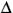x, each particle will move a distance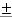x, moving right (+x) or left (-x) with equal probability.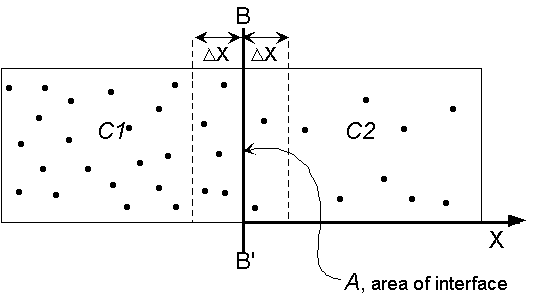Within each time step, any particle within a distanceX of the interface B-B' has a 50% probability of crossing over that interface. The number of particles with the potential to cross B-B' from left to right (positive mass flux) is (C1XA), where A is the area of interface B-B'.  On average half of these take a positive step and cross the interface in timet, such that the flux left to right is (0.5 C1x A).  Similarly, the number of particles crossing right to left int (negative mass flux) will be (0.5 C2X A).  The resulting mass flux, qX,, is

(1)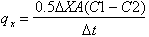.

If C(X) is continuous, then C2C1 +x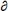C/x, and (1) becomes

(2)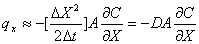.

D ~ (1/2)X2/t is the coefficient of diffusion, or diffusivity, and it units are [length2 time-1].   The diffusivity of a chemical molecule in a given fluid depends on the ease with which the molecule can move, specifically, how far,X, the molecule can move in a given time interval.  The ease of molecular motion, and thus the diffusivity of a particular chemical, will depend on the molecule size and polarity, the type of fluid and the temperature.

Equation (2) is a mathematical expression of Fick's Law.  Fick's Law states that the flux of solute mass crossing a unit area, A, per unit time,t, in a given direction, e.g. X, is proportional to the gradient of concentration in that direction,C/X, and is counter-gradient, i.e. the net flux is down-gradient.  Because the flux in any direction is proportional only to the concentration gradient in that direction, Fick's Law can be directly extended to three-dimensions.

(3)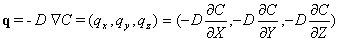.

Note that for molecular diffusion, considered here, the coefficient for diffusion is isotropic, i.e. the same in all directions.

## Diffusion from a point source

Consider a cloud of N particles (and total mass M) released at X = 0 and t = 0.  Under the action of molecular diffusion, the cloud will slowly spread.  We use the random walk model to predict the distribution of particle (mass) concentration , C(X,t).  Note, that if we assume a unit mass per particle, we can conveniently interchange N = M.  For simplicity we again consider a one-dimensional system, with the same rules of random motion described above, i.e. at each time step,t, each particle will move either +X or -X with equal probability. Over time each particle will move a bit forward and a bit backward.  The probable location of an individual particle after many such steps can be predicted with the Central Limit Theorem (see any basic statistics text).  Specifically, in the limit of many steps, the probability that a particle will be located between

mX and (m+1)X approaches a normal distribution with zero mean and a standard deviation of:

(4)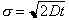,

where, as above,

(5)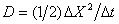.

The probability that a particle ends up between X and X+X is

(6).

Now consider the full cloud of N particles.  At any time t, the number of particles between X and X+X is expected to be n(X,t) =  N p(X,t)X.  Thus, the concentration, C, at position X is C(X,t) ~ n(X,t)/(AX), where A is the constant cross-section of the one-dimensional system.  Exchanging M for N, the concentration distribution, C(X,t), is

(7)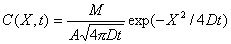=[mass / length3]

As noted above, this distribution is the normal distribution with zero mean and standard deviation,, which should be familiar to you from any basic statistics course.  Briefly, the distribution forms a bell-curve, as shown below.  At any time, sixty-eight percent of the total mass (total number of particles) falls within ±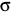of the mean position (X = 0).  Ninety-five percent of the mass falls within ± 2of the mean position.  And, 99.7% falls within ± 3of the mean position.  Based on these limits, it has become common to define the extent of a concentration patch based on the contour that includes ninety-five percent of the total mass, i.e. the contour at 2from the center.   With this convention the length of a diffusing cloud, L, is often taken as L = 4.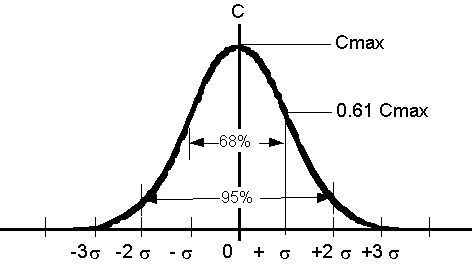It is also useful to note that the concentration level at one standard deviation from the cloud center is 61% of the maximum concentration, i.e. C (X =) = 0.61 Cmax, where Cmax is the concentration at the center of the cloud.  This value provides a useful way to rapidly define the standard deviation of a particle (species) cloud.

This animation shows the motion of 500 particles in a one-dimensional random walk with step sizeX = 1 in timet = 1.  At t = 0 the particles are located at X = 0.  This random walk animation mimics the effect of Fickian Diffusion.  As you watch the animation, consider the following.

Graph of Absolute Particle Location (lower left window).

This graph shows the number of particles located at each position of the X-axis.  The number of particles per location is analogous to a concentration.

1)      Over time how does the peak particle number, N, (concentration, C) change under the influence of random particle motion (diffusion)?

2)      Over time how does the gradient in particle number (concentration), i.e. dN/dX (dC/dX) change under the influence of random particle motion (diffusion)?

3)      What is the sign of the particle number (concentration) gradient (dN/dX) for X > 0? Consider the animation of individual particle motion (uppermost window).  For X > 0, is the net particle flux positive (to the right) or negative (to the left)?  Is the direction of flux up-gradient of down-gradient?  Is the relationship between the direction of flux and the concentration gradient consistent with Fick's Law (equation 3)?

4)      Estimate the diffusion coefficient, D, using equation (4) above, and the values ofgiven in the upper left corner of this graph.  Note that you can pause the animation.  How does the realized value of D compare with the theoretical value given in equation (5).  Note that no specific units are given here, such that D will simply have unit L2T-1, where L is an arbitrary length unit and T is the arbitrary time unit.

Graph of Particle Location in Terms of.

This graph plots the distribution of particle location with the position normalized by the standard deviation,.  The solid curve is the Gaussian distribution.

5)      Note that at early time (first few time steps) the real distribution of particles does not approximate a Gaussian distribution very well.  This is because the Gaussian distribution is only valid after a sufficient number of steps (Central Limit Theorem).  Use the animation to estimate how many steps are required for the distribution to consistently fit the Gaussian distribution.

## Conclusion - Key Aspects of Diffusion

(7)   Diffusion is the net flux due to random motion.

(8)   Diffusive flux is proportional but opposite in sign to the gradient of concentration.

(9)   Diffusion acts to dilute concentration and reduce gradients of concentration.